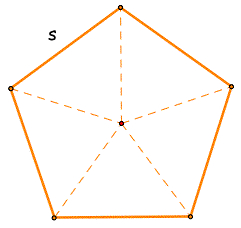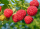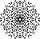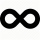# Of the 2

Of the shapes,1/6 are triangles and 5/12 are pentagons. What fraction of the shapes are either triangles or pentagons?

f =  0.5833

### Step-by-step explanation:Did you find an error or inaccuracy? Feel free to write us. Thank you!Tips to related online calculators
Need help to calculate sum, simplify or multiply fractions? Try our fraction calculator.

## Related math problems and questions:

• When 5When 5 is subtracted from both my numerator and denominator I become 3/4. What fraction i am?Nadia spent 1/4 of her money on a shirt and 2/5 of her money on new shoes. What fraction of Nadia´s money has been spent what fraction of her money is left?
• CarrieCarrie picked 2/5 of the raspberries from the garden, and Robin picked some too.  When they were finished, 1/3 of the raspberries still needed to be picked.  What fraction of the raspberries did Robin pick? Use pictures, numbers or words and write your fi
• DuringDuring a week, a student's spends 1/3 of her time in bed, 5/24 of her time in lesson and 1/8 of her time doing homeworks. What fraction of her time is left for doing other things?
• Simplify 3Simplify mixed numerals expression: 8 1/4- 3 2/5 - (2 1/3 - 1/4) Show your solution.
• Fractions and mixed numerals(a) Convert the following mixed numbers to improper fractions. i. 3 5/8 ii. 7 7/6 (b) Convert the following improper fraction to mixed number. i. 13/4 ii. 78/5 (c) Simplify these fractions to their lowest terms. i. 36/42 ii. 27/45 2. evaluate following ex
• FractionFind for what x fraction (-4x -6)/(x) equals:
• MichaelMichael had a bar of chocolate. He ate 1/2 of it and gave away 1/3. What fraction had he left?Mike buys flowers to plant around his trees. 3/8 of the flowers are red. 1/3 of the flowers are pink. The rest of the flowers are white. Find the fraction of flowers that are white.
• CupcakesIn a bowl was some cupcakes. Janka ate one third and Danka ate one quarter of cupcakes. a) How many of cookies ate together? b) How many cookies remain in a bowl? Write the results as a decimal number and in notepad also as a fraction.
• Sum of fractionsWhat is the sum of 2/3+3/5?
• What fractionWhat fraction of number 1 to 30 is prime?
• Division by zeroFraction 5 by 2. if 3 is added to numerator and 2 is subtracted from the denominator then the new fraction is:
• Length subtractingExpress in mm: 5 3/10 cm - 2/5 mm
• Sign of a expressionWhat is the sign of expression: minus 18 start fraction 9 divided by 17 end fraction plus left parenthesis minus 18 start fraction 9 divided by 17 end fraction right parenthesis?
• Evaluate 17Evaluate 2x+6y when x=- 4/5 and y=1/3. Write your answer as a fraction or mixed number in simplest form.
• CompareCompare fractions (34)/(3) and (12)/(4). Which fraction of the lower?